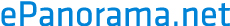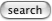# How does VU and dB meters differ ?

VU and dB meters both measure the audio power involved in recording and they both use logarithmic scales to report that power. Because of these logarithmic scales, a factor of 10 increase in power produces an increase of 10 in both the VU reading and the dB reading. For example, -20 dB is 10 times the power of -30 dB. In both measures, the zero is chosen as the highest acceptable power--the highest power for which distortion is acceptible. Where VU and dB differ is in how they measure audio power.

VU is short for "volume units" and it is a measure of average audio power. A VU meter responds relatively slowly and considers the sound volume over a period of time. Its zero is set to the level at which there is 1% total harmonic distortion in the recorded signal.

dB is short for "decibels" and it is a measure of instantaneous audio power. A dB meter responds very rapidly and considers the audio power at each instant. Its zero is set to the level at which there is 3% total harmonic distortion.

Because of these differences in zero definitions, the dB meter's zero is roughly at the VU meter's +8. Nonetheless, both meters are important and both should be kept at or below zero to avoid significant distortion in a recording. In certain situations, such as when there are sudden loud sounds or with instruments that are very rich in harmonics, it's possible to have the dB meter read above zero even though the VU meter remains below zero.